# Pascal Triangle

11. Jul 2014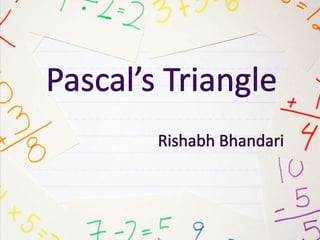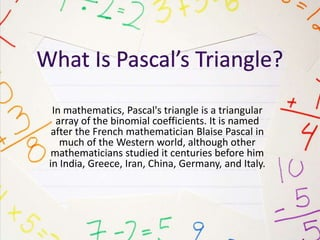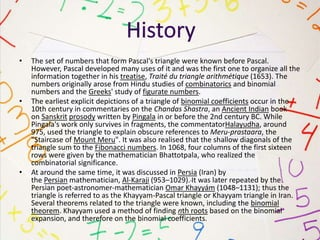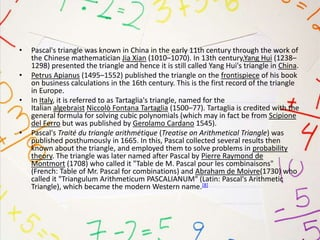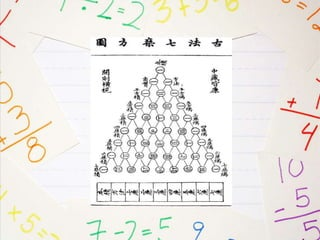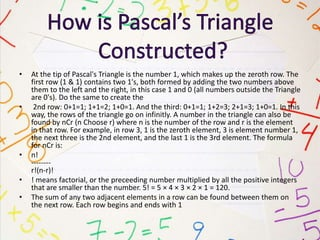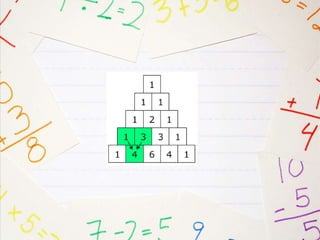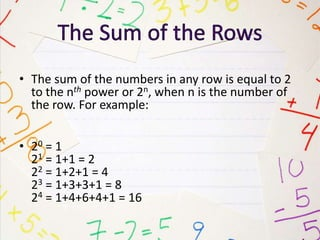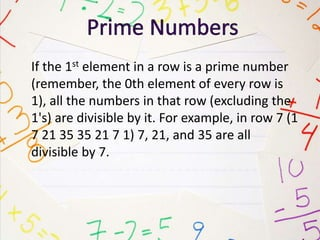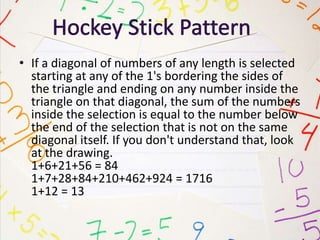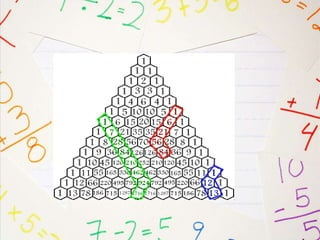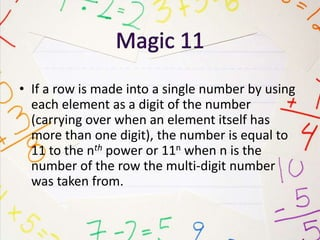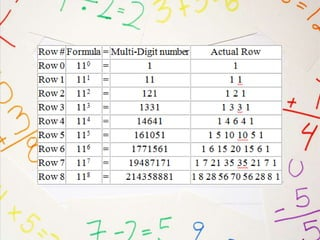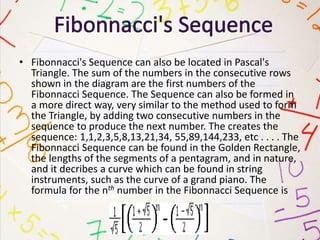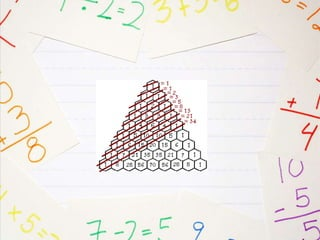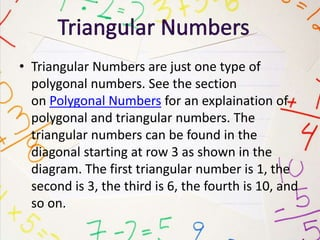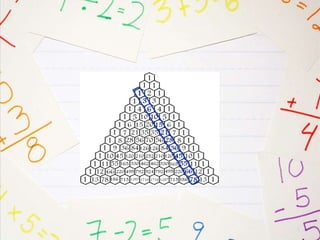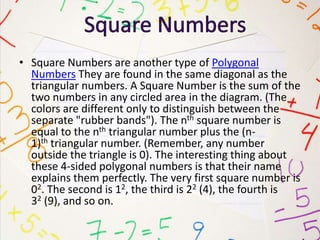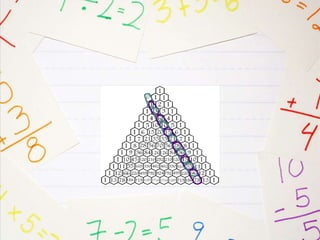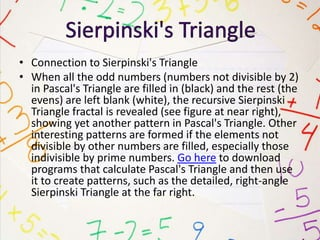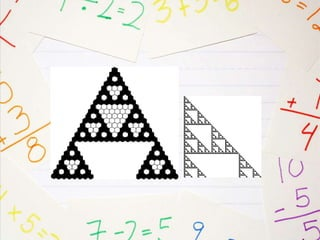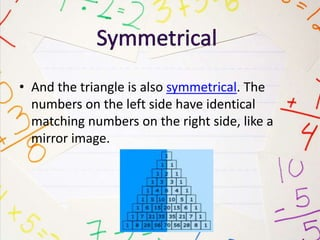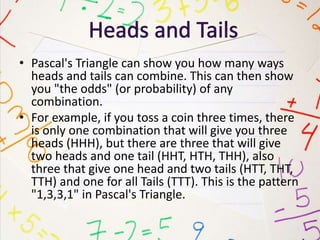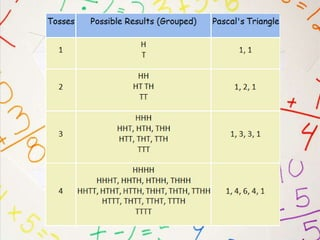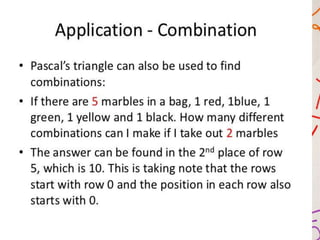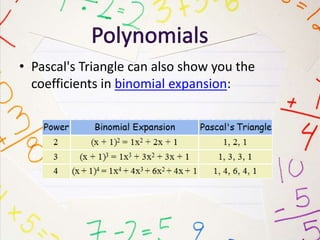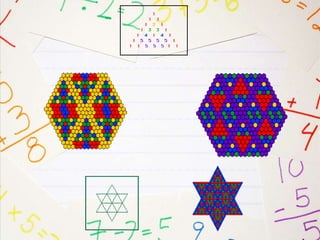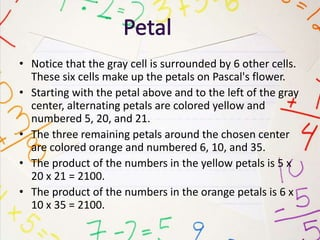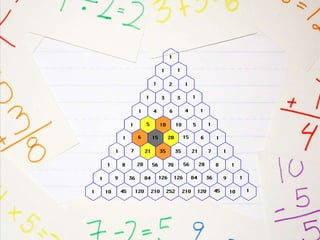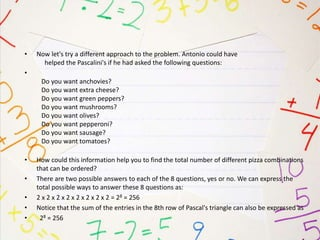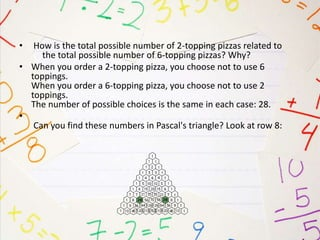1 von 36

### Pascal Triangle

• 2. In mathematics, Pascal's triangle is a triangular array of the binomial coefficients. It is named after the French mathematician Blaise Pascal in much of the Western world, although other mathematicians studied it centuries before him in India, Greece, Iran, China, Germany, and Italy.
• 3. • The set of numbers that form Pascal's triangle were known before Pascal. However, Pascal developed many uses of it and was the first one to organize all the information together in his treatise, Traité du triangle arithmétique (1653). The numbers originally arose from Hindu studies of combinatorics and binomial numbers and the Greeks' study of figurate numbers. • The earliest explicit depictions of a triangle of binomial coefficients occur in the 10th century in commentaries on the Chandas Shastra, an Ancient Indian book on Sanskrit prosody written by Pingala in or before the 2nd century BC. While Pingala's work only survives in fragments, the commentatorHalayudha, around 975, used the triangle to explain obscure references to Meru-prastaara, the "Staircase of Mount Meru". It was also realised that the shallow diagonals of the triangle sum to the Fibonacci numbers. In 1068, four columns of the first sixteen rows were given by the mathematician Bhattotpala, who realized the combinatorial significance. • At around the same time, it was discussed in Persia (Iran) by the Persian mathematician, Al-Karaji (953–1029). It was later repeated by the Persian poet-astronomer-mathematician Omar Khayyám (1048–1131); thus the triangle is referred to as the Khayyam-Pascal triangle or Khayyam triangle in Iran. Several theorems related to the triangle were known, including the binomial theorem. Khayyam used a method of finding nth roots based on the binomial expansion, and therefore on the binomial coefficients.
• 4. • Pascal's triangle was known in China in the early 11th century through the work of the Chinese mathematician Jia Xian (1010–1070). In 13th century,Yang Hui (1238– 1298) presented the triangle and hence it is still called Yang Hui's triangle in China. • Petrus Apianus (1495–1552) published the triangle on the frontispiece of his book on business calculations in the 16th century. This is the first record of the triangle in Europe. • In Italy, it is referred to as Tartaglia's triangle, named for the Italian algebraist Niccolò Fontana Tartaglia (1500–77). Tartaglia is credited with the general formula for solving cubic polynomials (which may in fact be from Scipione del Ferro but was published by Gerolamo Cardano 1545). • Pascal's Traité du triangle arithmétique (Treatise on Arithmetical Triangle) was published posthumously in 1665. In this, Pascal collected several results then known about the triangle, and employed them to solve problems in probability theory. The triangle was later named after Pascal by Pierre Raymond de Montmort (1708) who called it "Table de M. Pascal pour les combinaisons" (French: Table of Mr. Pascal for combinations) and Abraham de Moivre(1730) who called it "Triangulum Arithmeticum PASCALIANUM" (Latin: Pascal's Arithmetic Triangle), which became the modern Western name.
• 6. • At the tip of Pascal's Triangle is the number 1, which makes up the zeroth row. The first row (1 & 1) contains two 1's, both formed by adding the two numbers above them to the left and the right, in this case 1 and 0 (all numbers outside the Triangle are 0's). Do the same to create the • 2nd row: 0+1=1; 1+1=2; 1+0=1. And the third: 0+1=1; 1+2=3; 2+1=3; 1+0=1. In this way, the rows of the triangle go on infinitly. A number in the triangle can also be found by nCr (n Choose r) where n is the number of the row and r is the element in that row. For example, in row 3, 1 is the zeroth element, 3 is element number 1, the next three is the 2nd element, and the last 1 is the 3rd element. The formula for nCr is: • n! -------- r!(n-r)! • ! means factorial, or the preceeding number multiplied by all the positive integers that are smaller than the number. 5! = 5 × 4 × 3 × 2 × 1 = 120. • The sum of any two adjacent elements in a row can be found between them on the next row. Each row begins and ends with 1
• 8. • The sum of the numbers in any row is equal to 2 to the nth power or 2n, when n is the number of the row. For example: • 20 = 1 21 = 1+1 = 2 22 = 1+2+1 = 4 23 = 1+3+3+1 = 8 24 = 1+4+6+4+1 = 16
• 9. If the 1st element in a row is a prime number (remember, the 0th element of every row is 1), all the numbers in that row (excluding the 1's) are divisible by it. For example, in row 7 (1 7 21 35 35 21 7 1) 7, 21, and 35 are all divisible by 7.
• 10. • If a diagonal of numbers of any length is selected starting at any of the 1's bordering the sides of the triangle and ending on any number inside the triangle on that diagonal, the sum of the numbers inside the selection is equal to the number below the end of the selection that is not on the same diagonal itself. If you don't understand that, look at the drawing. 1+6+21+56 = 84 1+7+28+84+210+462+924 = 1716 1+12 = 13
• 12. • If a row is made into a single number by using each element as a digit of the number (carrying over when an element itself has more than one digit), the number is equal to 11 to the nth power or 11n when n is the number of the row the multi-digit number was taken from.
• 14. • Fibonnacci's Sequence can also be located in Pascal's Triangle. The sum of the numbers in the consecutive rows shown in the diagram are the first numbers of the Fibonnacci Sequence. The Sequence can also be formed in a more direct way, very similar to the method used to form the Triangle, by adding two consecutive numbers in the sequence to produce the next number. The creates the sequence: 1,1,2,3,5,8,13,21,34, 55,89,144,233, etc . . . . The Fibonnacci Sequence can be found in the Golden Rectangle, the lengths of the segments of a pentagram, and in nature, and it decribes a curve which can be found in string instruments, such as the curve of a grand piano. The formula for the nth number in the Fibonnacci Sequence is
• 16. • Triangular Numbers are just one type of polygonal numbers. See the section on Polygonal Numbers for an explaination of polygonal and triangular numbers. The triangular numbers can be found in the diagonal starting at row 3 as shown in the diagram. The first triangular number is 1, the second is 3, the third is 6, the fourth is 10, and so on.
• 18. • Square Numbers are another type of Polygonal Numbers They are found in the same diagonal as the triangular numbers. A Square Number is the sum of the two numbers in any circled area in the diagram. (The colors are different only to distinguish between the separate "rubber bands"). The nth square number is equal to the nth triangular number plus the (n- 1)th triangular number. (Remember, any number outside the triangle is 0). The interesting thing about these 4-sided polygonal numbers is that their name explains them perfectly. The very first square number is 02. The second is 12, the third is 22 (4), the fourth is 32 (9), and so on.
• 20. • Connection to Sierpinski's Triangle • When all the odd numbers (numbers not divisible by 2) in Pascal's Triangle are filled in (black) and the rest (the evens) are left blank (white), the recursive Sierpinski Triangle fractal is revealed (see figure at near right), showing yet another pattern in Pascal's Triangle. Other interesting patterns are formed if the elements not divisible by other numbers are filled, especially those indivisible by prime numbers. Go here to download programs that calculate Pascal's Triangle and then use it to create patterns, such as the detailed, right-angle Sierpinski Triangle at the far right.
• 22. • And the triangle is also symmetrical. The numbers on the left side have identical matching numbers on the right side, like a mirror image.
• 24. • Pascal's Triangle can show you how many ways heads and tails can combine. This can then show you "the odds" (or probability) of any combination. • For example, if you toss a coin three times, there is only one combination that will give you three heads (HHH), but there are three that will give two heads and one tail (HHT, HTH, THH), also three that give one head and two tails (HTT, THT, TTH) and one for all Tails (TTT). This is the pattern "1,3,3,1" in Pascal's Triangle.
• 27. • Pascal's Triangle can also show you the coefficients in binomial expansion:
• 28. • Choose any five colors. Assign a different color to each number and shade each block on the color chart accordingly. (See closure, below.) • • Print a blank Pascal Triangle grid from the student worksheets page.Color the top three hexagons color 1. (Using black for color 1 provides a nice outline.) • 4. To determine the color of the next row of cells, look at the last row: • if there is only one cell above a cell, make that cell color 1. • if there are two cells above a cell, use the chart to find the color to use. • if the two cells above are both color 1, look at row 1 of column 1 on the chart for the color to use. It is color 2. • if the two cells above are colors 1 and 2, look at row 1 of column 2: it tells you to use color 3.
• 30. • Notice that the gray cell is surrounded by 6 other cells. These six cells make up the petals on Pascal's flower. • Starting with the petal above and to the left of the gray center, alternating petals are colored yellow and numbered 5, 20, and 21. • The three remaining petals around the chosen center are colored orange and numbered 6, 10, and 35. • The product of the numbers in the yellow petals is 5 x 20 x 21 = 2100. • The product of the numbers in the orange petals is 6 x 10 x 35 = 2100.
• 32. • How many different 1-topping pizzas can you order when choosing from among 8 toppings? • • You can order 8 different 1-topping pizzas • You can find the answer by listing the 8 possible pizzas, as shown above, or think: how many different pizza combinations can I make by choosing 1 topping from a set of 8 toppings? • Using Pascal's triangle, find place 1 in row 8: 8 ways. [Remember that the first number (1) in each row is place 0.]
• 33. • Now let's try a different approach to the problem. Antonio could have helped the Pascalini's if he had asked the following questions: • Do you want anchovies? Do you want extra cheese? Do you want green peppers? Do you want mushrooms? Do you want olives? Do you want pepperoni? Do you want sausage? Do you want tomatoes? • How could this information help you to find the total number of different pizza combinations that can be ordered? • There are two possible answers to each of the 8 questions, yes or no. We can express the total possible ways to answer these 8 questions as: • 2 x 2 x 2 x 2 x 2 x 2 x 2 x 2 = 28 = 256 • Notice that the sum of the entries in the 8th row of Pascal's triangle can also be expressed as • 28 = 256
• 34. • How many different 7-topping pizza combinations can you order from a set of 8 toppings? • You can order 8 different 7-topping pizzas: You can find this answer by listing the 8 possible pizzas, as shown above, or think: how many different 7-topping pizza combinations can I make from a set of 8 toppings? Using Pascal's triangle, find place 7 in row 8: 8 ways.
• 35. • How many different pizza combinations can you make using 2 toppings? • You can order 28 different pizza combinations when you choose 2 toppings from a set of 8 toppings
• 36. • How is the total possible number of 2-topping pizzas related to the total possible number of 6-topping pizzas? Why? • When you order a 2-topping pizza, you choose not to use 6 toppings. When you order a 6-topping pizza, you choose not to use 2 toppings. The number of possible choices is the same in each case: 28. • Can you find these numbers in Pascal's triangle? Look at row 8: